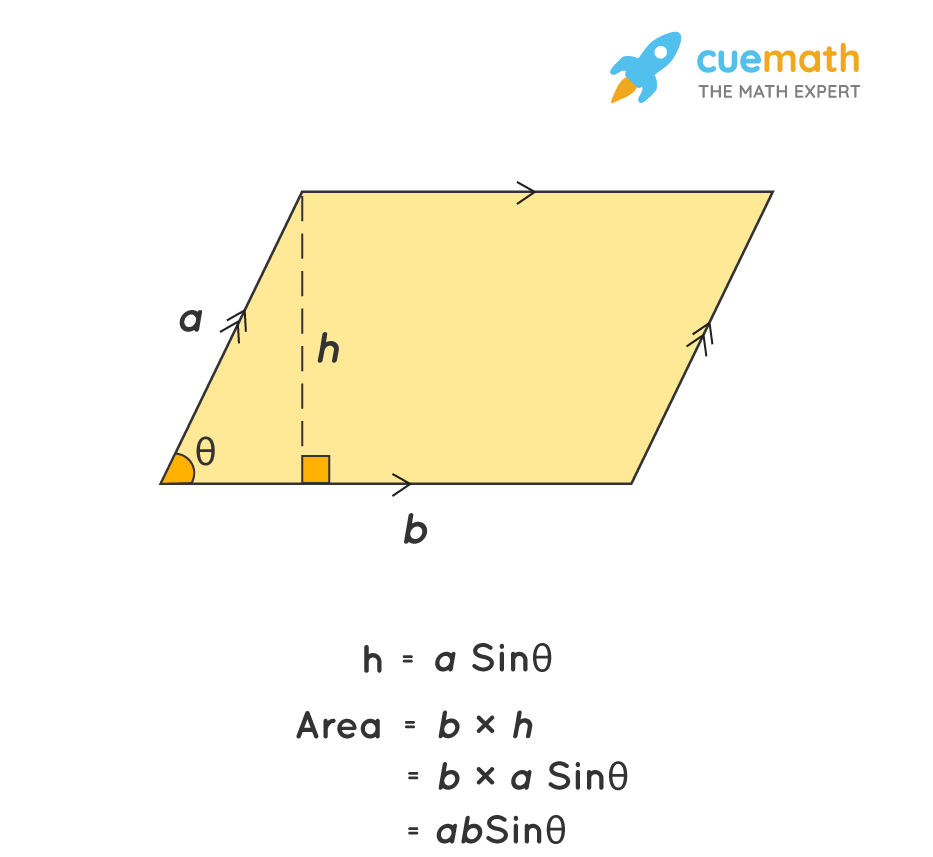# Area of a Parallelogram With Sides of 6 and 12 and an Angle of 60°.

## Question: Find the area of a parallelogram with sides of 6 and 12 and an angle of 60°.

The area of a parallelogram is base × height.

## Answer: The area of a parallelogram with sides of 6 and 12 and an angle of 60° is 36√3 = 62.3538 square units.

Let us find the area of a parallelogram with the given parameters.

## Explanation:

The area of a parallelogram is b × h = b × a sinθ = absinθ.Area = absinθ

Area = 6 × 12 × sin(60°)

Area = 6 × 12 × (√3/2)

Area = 36√3 = 62.3538 sq. units